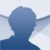SageMath

8.9 v0.5.1

SageMath is an advanced maths calculation software that can be used in academic fields and that is supposed to be a decent Matlab or Maple alternative

When it comes to teaching and learning maths, SageMath is one of the best alternatives to Matlab and other similar software. It's an open-source development which makes it perfect for the academic scope and can be applied to both basic and advanced maths, whether pure or applied mathematics.

Created by mathematicians

This program has been developed by mathematicians and includes loads of functions that cover all sorts of branches: algebra, calculus (elemental and advanced), arithmetics, combinatorial analysis, geometry... and it does so thanks to different software packages to integrate each function into its interface.

It's used by plenty of users within the educational community, therefore, it's not hard to find tutorials about how it works, both on video or downloadable PDFs, covering all sorts of actions that can be developed with this software. It has been developed with Python and runs in a browser configured for such purpose on our PC. It brings together several different tools on the same interface, and displayed hierarchically, for the following fields:

• Algebra.
• Linear algebra.
• Arbitrary-precision arithmetic.
• Calculus.
• Combinatorial analysis.
• Statistics.
• Algebraic geometry.
• Arithmetic geometry.
• Differential geometry and Tensor Calculus.
• Graph Theory.
• Group Theory.
• Number Theory.

We also have to point out that it provides users with a method to combine software and compare results with non-free programs such as Mathematica, Maple, and Magma.

SageMath
Over a year ago
More than a year ago
864 MB

We'd love to hear from you. Do you want to give us your opinion?Logged off